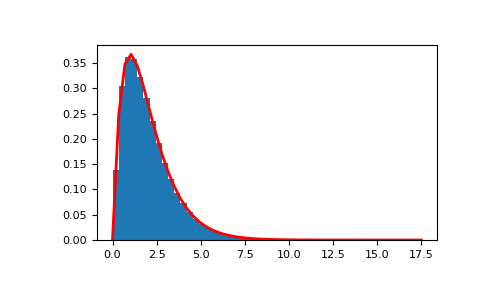#### Previous topic

numpy.random.standard_exponential

#### Next topic

numpy.random.standard_normal

# numpy.random.standard_gamma¶

numpy.random.standard_gamma(shape, size=None)

Draw samples from a standard Gamma distribution.

Samples are drawn from a Gamma distribution with specified parameters, shape (sometimes designated “k”) and scale=1.

Note

New code should use the standard_gamma method of a default_rng() instance instead; see random-quick-start.

Parameters
shapefloat or array_like of floats

Parameter, must be non-negative.

sizeint or tuple of ints, optional

Output shape. If the given shape is, e.g., (m, n, k), then m * n * k samples are drawn. If size is None (default), a single value is returned if shape is a scalar. Otherwise, np.array(shape).size samples are drawn.

Returns
outndarray or scalar

Drawn samples from the parameterized standard gamma distribution.

scipy.stats.gamma

probability density function, distribution or cumulative density function, etc.

Generator.standard_gamma

which should be used for new code.

Notes

The probability density for the Gamma distribution iswhereis the shape andthe scale, andis the Gamma function.

The Gamma distribution is often used to model the times to failure of electronic components, and arises naturally in processes for which the waiting times between Poisson distributed events are relevant.

References

1

Weisstein, Eric W. “Gamma Distribution.” From MathWorld–A Wolfram Web Resource. http://mathworld.wolfram.com/GammaDistribution.html

2

Wikipedia, “Gamma distribution”, https://en.wikipedia.org/wiki/Gamma_distribution

Examples

Draw samples from the distribution:

>>> shape, scale = 2., 1. # mean and width
>>> s = np.random.standard_gamma(shape, 1000000)


Display the histogram of the samples, along with the probability density function:

>>> import matplotlib.pyplot as plt
>>> import scipy.special as sps
>>> count, bins, ignored = plt.hist(s, 50, density=True)
>>> y = bins**(shape-1) * ((np.exp(-bins/scale))/
...                       (sps.gamma(shape) * scale**shape))
>>> plt.plot(bins, y, linewidth=2, color='r')
>>> plt.show()